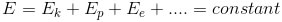﻿ All the formulas related to Work And Energy

# List of formulas related to Work and energy

#### Work

W: work done by a force (j), F: force move a object for a distance s, α: angle between F and movement direction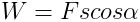#### Example

Wp: work by gravity, h: the change in height; wms:work of friction’s force; W: work by a spring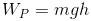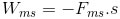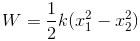#### Power

P: power (watt, W), t: time of conducting the work W(s)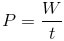#### Kinetic Energy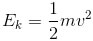#### Kinetic Energy Of A Massive Rotating Body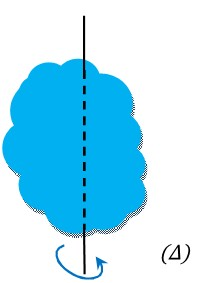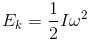#### Work Energy Principle

v1, v2: speeds of object before and after the change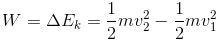#### Potential Energy

Ep: gravitational potential energy (j), m: mass of object (kg)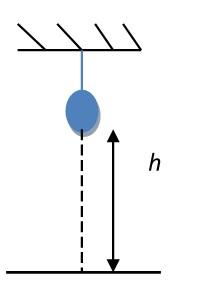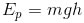#### Conservation of Mechanical Energy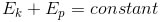#### Conservation Of Energy